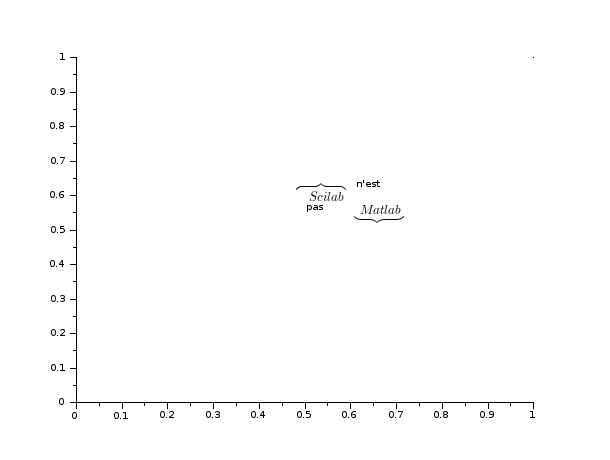Change language to:
Français - 日本語 - Português - Русский

See the recommended documentation of this function

Scilab help >> Graphics > text > xstring

# xstring

draw strings

### Calling Sequence

`xstring(x,y,str,[angle,[box]])`

### Arguments

x,y

real vectors or scalars, coordinates of the lower-left point of the strings.

str

matrix of strings.

Starting from Scilab 5.2, it is possible to write LaTeX or MathML expression.

angle

real vector or scalar, clockwise angle in degree; default is 0.

box

integer vector or scalar, default is 0.

### Description

If `x` is a real scalar, it's regarded as a vector with the size of `y` whose elements are set to `x`.

If `y` is a real scalar, it's regarded as a vector with the size of `x` whose elements are set to `y`.

`xstring` draws n strings at location `(x[i], y[i])` in the current graphic scale.

If `str` contain n elements, these n elements are the n drawed strings.

Otherwise, each row of the matrix stands for a line of text and row elements stand for words separated by a white space.

If `angle` is a real scalar, it's regarded as a vector of size n whose elements are set to `angle`.

`angle(i)`gives the slope in degree used for drawing the strings at location `(x[i], y[i])`.

If `box` is an integer scalar, it's regarded as a vector of size n whose elements are set to `box`.

If `box(i)` is 1 and `angle(i)` is 0, a box is drawn around the strings at location `(x[i], y[i])`.

### Examples

```plot2d([0;1],[0;1],0)
xstring(0.5,0.5,["\$\overbrace{Scilab}\$" "n''est ";"pas" "\$\underbrace{Matlab}\$"])
// LaTeX rendering (>= Scilab 5.2)

//Other example
alphabet=["a" "b" "c" "d" "e" "f" "g" ..
"h" "i" "j" "k" "l" "m" "n" ..
"o" "p" "q" "r" "s" "t" "u" ..
"v" "w" "x" "y" "z"];
clf()
plot2d([0;1],[0;2],0)
xstring(0.1,1.8,alphabet)     // alphabet
xstring(0.1,1.6,alphabet,0,1) // alphabet in a box
xstring(0.1,1.4,alphabet,20)  // angle
xset("font",1,1)              // use symbol fonts
xstring(0.1,0.1,alphabet)
xset("font",1,3)              // change size font
xstring(0.1,0.3,alphabet)
xset("font",1,24);
xstring(0.1,0.6,"a") //big alpha```